Скачать презентацию CMSC 471 First-Order Logic Chapter 8 1 -8

330867942be2b75908650133ba91bf2d.ppt

• Количество слайдов: 50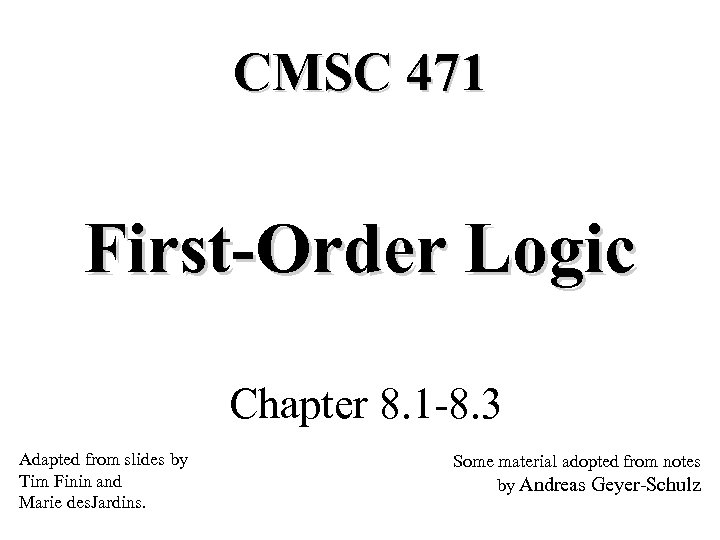CMSC 471 First-Order Logic Chapter 8. 1 -8. 3 Adapted from slides by Tim Finin and Marie des. Jardins. Some material adopted from notes by Andreas Geyer-Schulz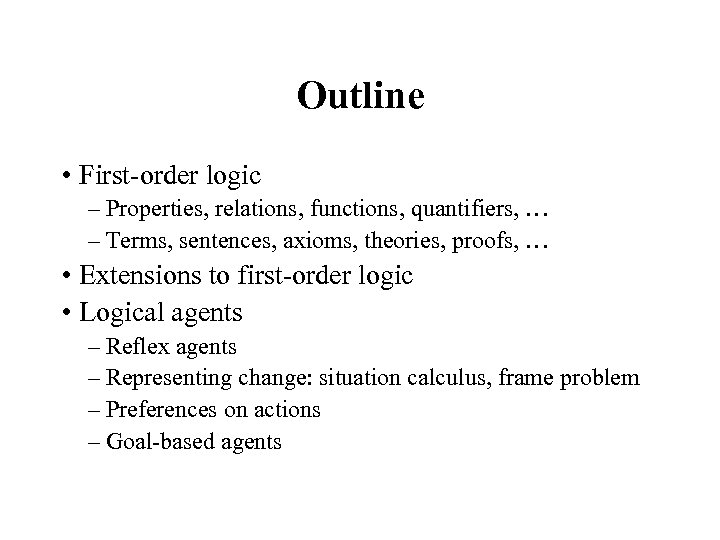Outline • First-order logic – Properties, relations, functions, quantifiers, … – Terms, sentences, axioms, theories, proofs, … • Extensions to first-order logic • Logical agents – Reflex agents – Representing change: situation calculus, frame problem – Preferences on actions – Goal-based agents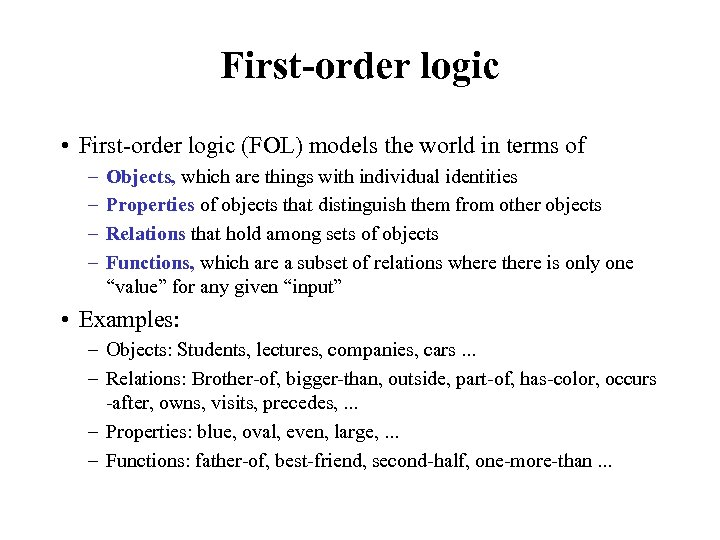First-order logic • First-order logic (FOL) models the world in terms of – – Objects, which are things with individual identities Properties of objects that distinguish them from other objects Relations that hold among sets of objects Functions, which are a subset of relations where there is only one “value” for any given “input” • Examples: – Objects: Students, lectures, companies, cars. . . – Relations: Brother-of, bigger-than, outside, part-of, has-color, occurs -after, owns, visits, precedes, . . . – Properties: blue, oval, even, large, . . . – Functions: father-of, best-friend, second-half, one-more-than. . .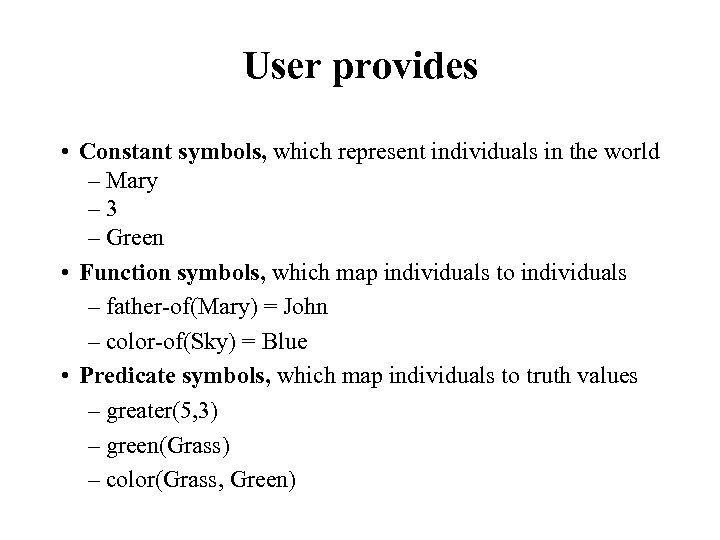User provides • Constant symbols, which represent individuals in the world – Mary – 3 – Green • Function symbols, which map individuals to individuals – father-of(Mary) = John – color-of(Sky) = Blue • Predicate symbols, which map individuals to truth values – greater(5, 3) – green(Grass) – color(Grass, Green)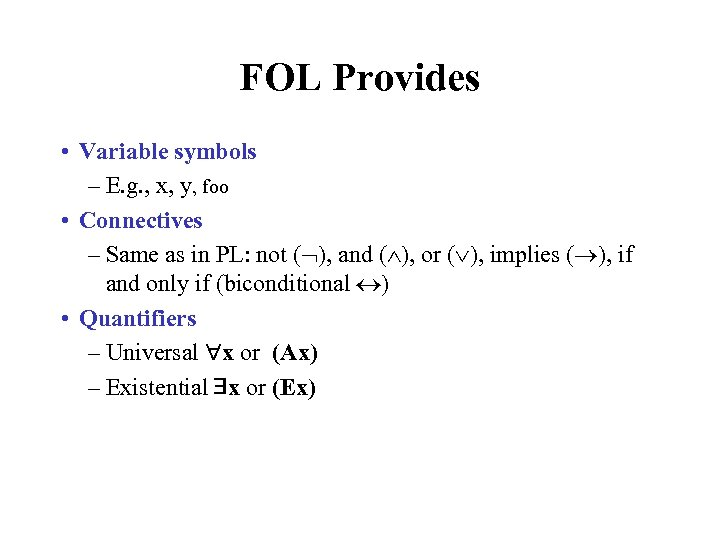FOL Provides • Variable symbols – E. g. , x, y, foo • Connectives – Same as in PL: not ( ), and ( ), or ( ), implies ( ), if and only if (biconditional ) • Quantifiers – Universal x or (Ax) – Existential x or (Ex)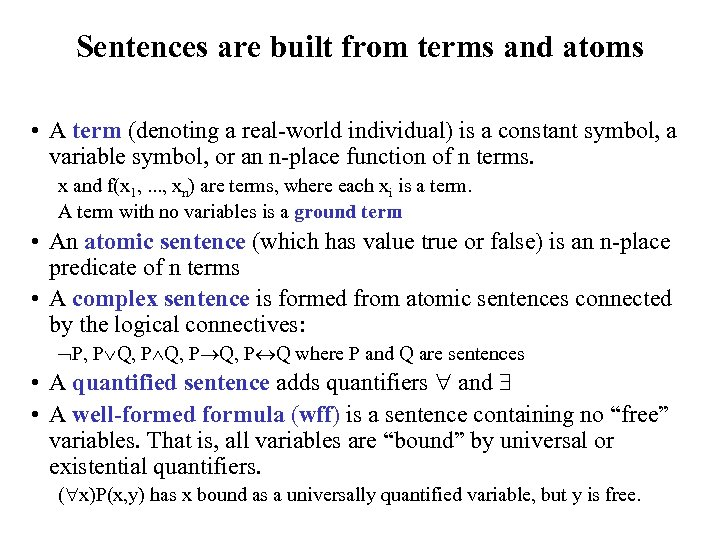Sentences are built from terms and atoms • A term (denoting a real-world individual) is a constant symbol, a variable symbol, or an n-place function of n terms. x and f(x 1, . . . , xn) are terms, where each xi is a term. A term with no variables is a ground term • An atomic sentence (which has value true or false) is an n-place predicate of n terms • A complex sentence is formed from atomic sentences connected by the logical connectives: P, P Q, P Q where P and Q are sentences • A quantified sentence adds quantifiers and • A well-formed formula (wff) is a sentence containing no “free” variables. That is, all variables are “bound” by universal or existential quantifiers. ( x)P(x, y) has x bound as a universally quantified variable, but y is free.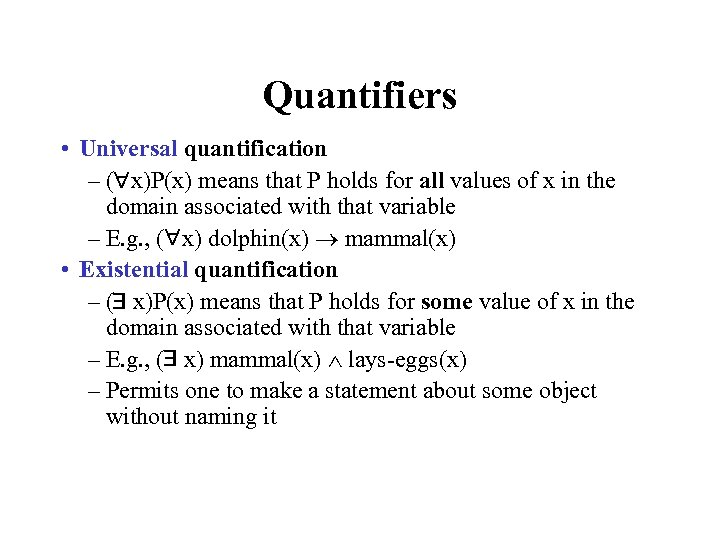Quantifiers • Universal quantification – ( x)P(x) means that P holds for all values of x in the domain associated with that variable – E. g. , ( x) dolphin(x) mammal(x) • Existential quantification – ( x)P(x) means that P holds for some value of x in the domain associated with that variable – E. g. , ( x) mammal(x) lays-eggs(x) – Permits one to make a statement about some object without naming it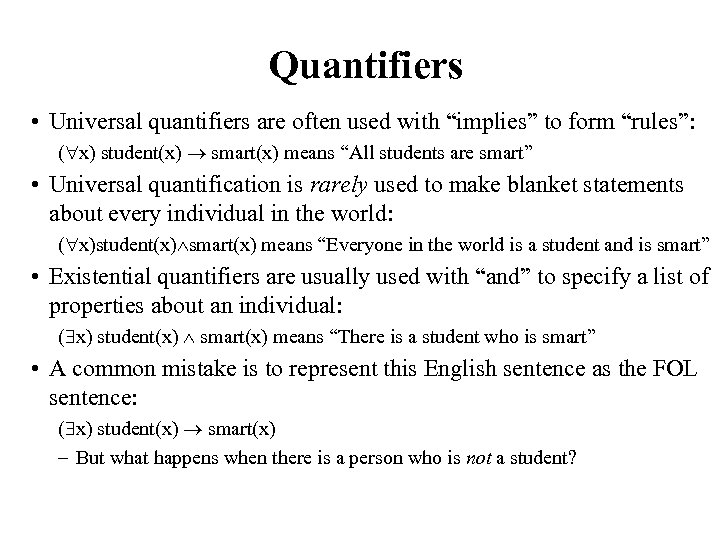Quantifiers • Universal quantifiers are often used with “implies” to form “rules”: ( x) student(x) smart(x) means “All students are smart” • Universal quantification is rarely used to make blanket statements about every individual in the world: ( x)student(x) smart(x) means “Everyone in the world is a student and is smart” • Existential quantifiers are usually used with “and” to specify a list of properties about an individual: ( x) student(x) smart(x) means “There is a student who is smart” • A common mistake is to represent this English sentence as the FOL sentence: ( x) student(x) smart(x) – But what happens when there is a person who is not a student?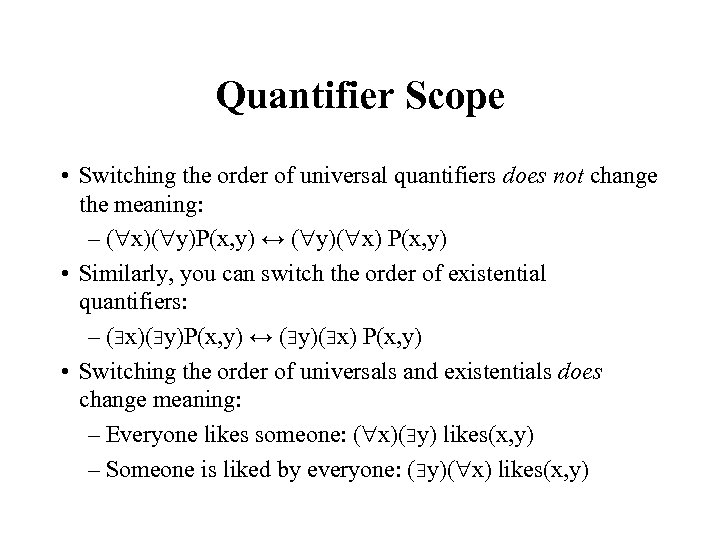Quantifier Scope • Switching the order of universal quantifiers does not change the meaning: – ( x)( y)P(x, y) ↔ ( y)( x) P(x, y) • Similarly, you can switch the order of existential quantifiers: – ( x)( y)P(x, y) ↔ ( y)( x) P(x, y) • Switching the order of universals and existentials does change meaning: – Everyone likes someone: ( x)( y) likes(x, y) – Someone is liked by everyone: ( y)( x) likes(x, y)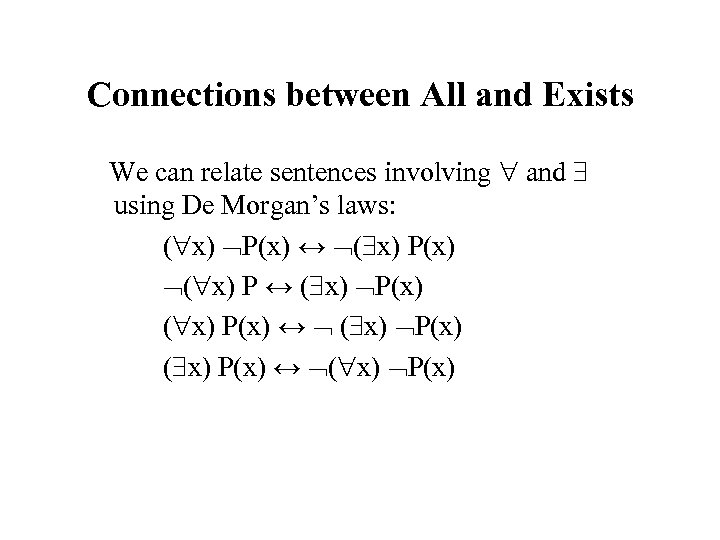Connections between All and Exists We can relate sentences involving and using De Morgan’s laws: ( x) P(x) ↔ ( x) P(x) ↔ ( x) P(x) ↔ ( x) P(x)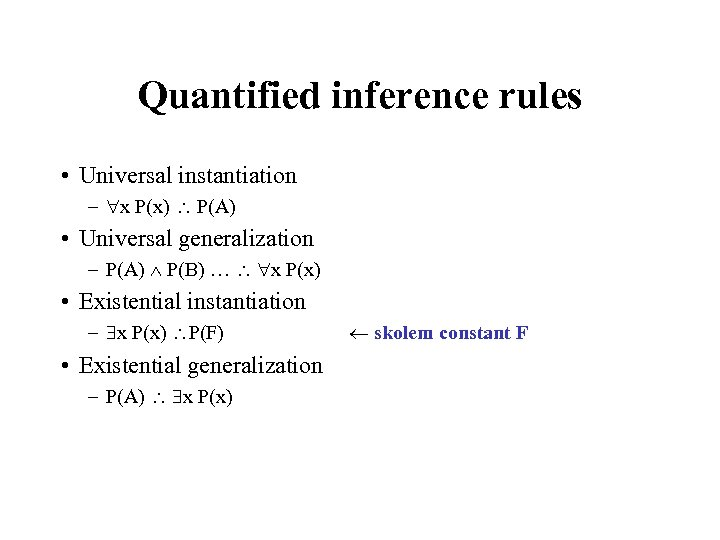Quantified inference rules • Universal instantiation – x P(x) P(A) • Universal generalization – P(A) P(B) … x P(x) • Existential instantiation – x P(x) P(F) • Existential generalization – P(A) x P(x) skolem constant F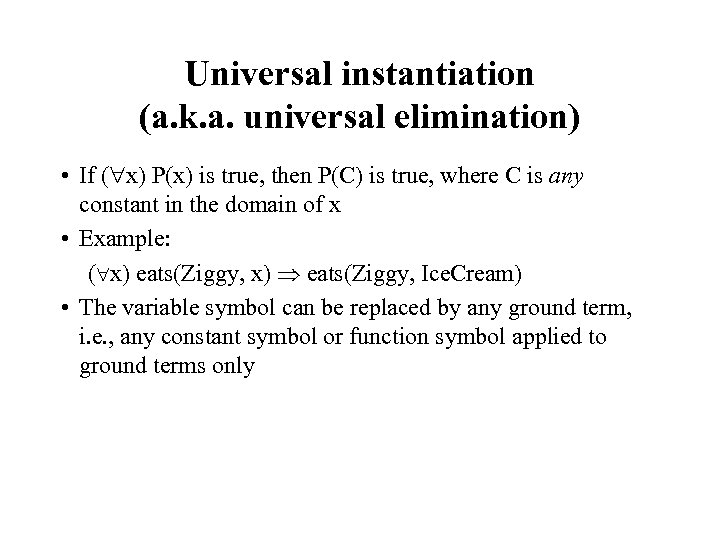Universal instantiation (a. k. a. universal elimination) • If ( x) P(x) is true, then P(C) is true, where C is any constant in the domain of x • Example: ( x) eats(Ziggy, x) eats(Ziggy, Ice. Cream) • The variable symbol can be replaced by any ground term, i. e. , any constant symbol or function symbol applied to ground terms only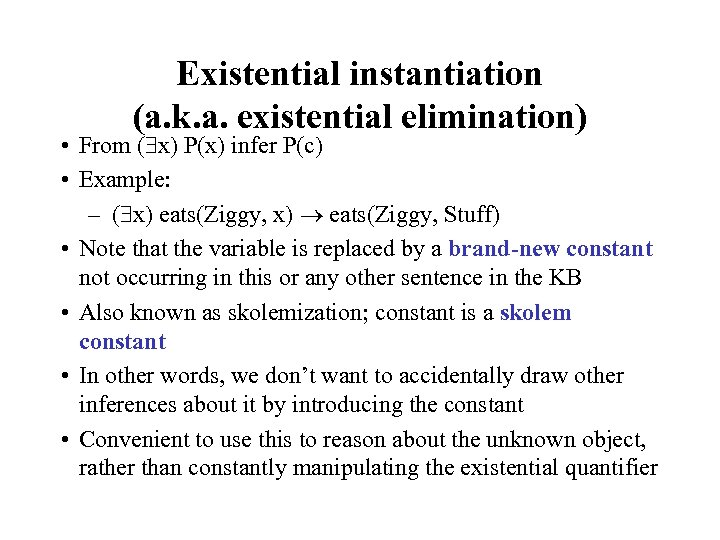Existential instantiation (a. k. a. existential elimination) • From ( x) P(x) infer P(c) • Example: – ( x) eats(Ziggy, x) eats(Ziggy, Stuff) • Note that the variable is replaced by a brand-new constant not occurring in this or any other sentence in the KB • Also known as skolemization; constant is a skolem constant • In other words, we don’t want to accidentally draw other inferences about it by introducing the constant • Convenient to use this to reason about the unknown object, rather than constantly manipulating the existential quantifier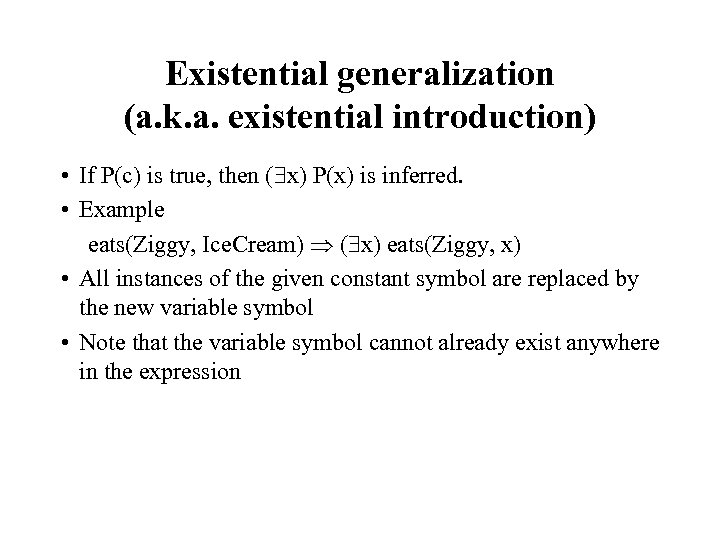Existential generalization (a. k. a. existential introduction) • If P(c) is true, then ( x) P(x) is inferred. • Example eats(Ziggy, Ice. Cream) ( x) eats(Ziggy, x) • All instances of the given constant symbol are replaced by the new variable symbol • Note that the variable symbol cannot already exist anywhere in the expression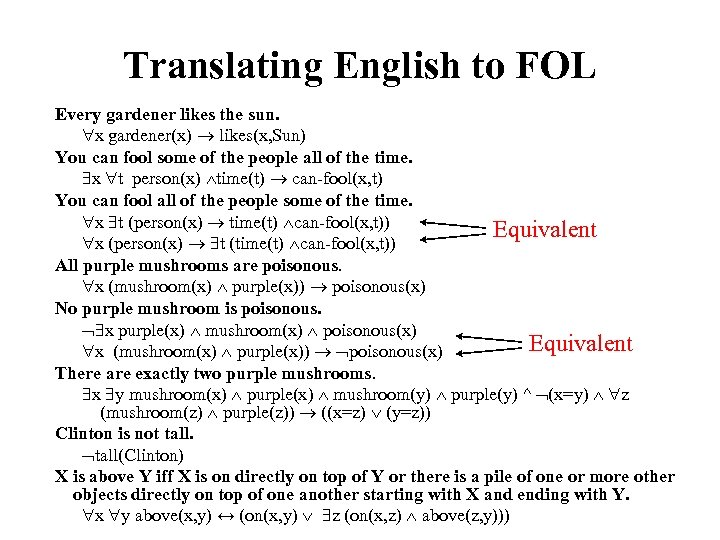Translating English to FOL Every gardener likes the sun. x gardener(x) likes(x, Sun) You can fool some of the people all of the time. x t person(x) time(t) can-fool(x, t) You can fool all of the people some of the time. x t (person(x) time(t) can-fool(x, t)) Equivalent x (person(x) t (time(t) can-fool(x, t)) All purple mushrooms are poisonous. x (mushroom(x) purple(x)) poisonous(x) No purple mushroom is poisonous. x purple(x) mushroom(x) poisonous(x) Equivalent x (mushroom(x) purple(x)) poisonous(x) There are exactly two purple mushrooms. x y mushroom(x) purple(x) mushroom(y) purple(y) ^ (x=y) z (mushroom(z) purple(z)) ((x=z) (y=z)) Clinton is not tall(Clinton) X is above Y iff X is on directly on top of Y or there is a pile of one or more other objects directly on top of one another starting with X and ending with Y. x y above(x, y) ↔ (on(x, y) z (on(x, z) above(z, y)))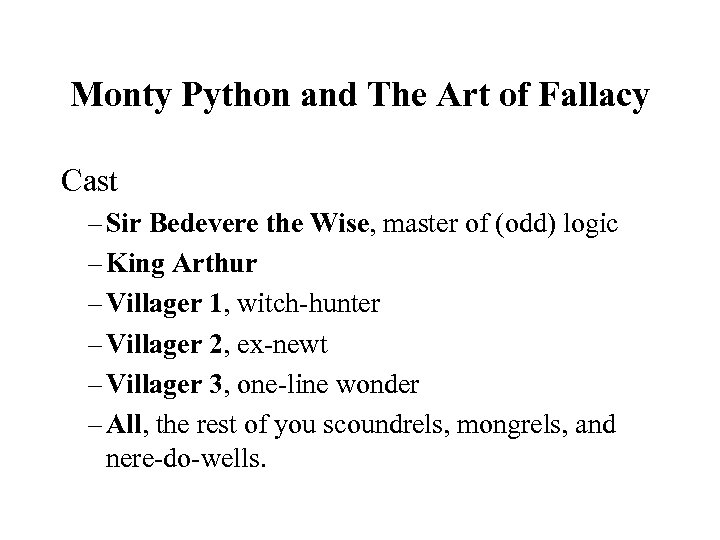Monty Python and The Art of Fallacy Cast – Sir Bedevere the Wise, master of (odd) logic – King Arthur – Villager 1, witch-hunter – Villager 2, ex-newt – Villager 3, one-line wonder – All, the rest of you scoundrels, mongrels, and nere-do-wells.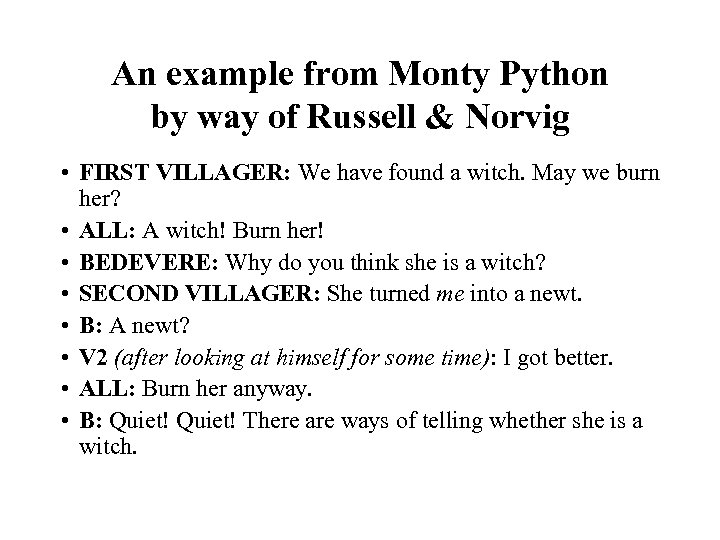An example from Monty Python by way of Russell & Norvig • FIRST VILLAGER: We have found a witch. May we burn her? • ALL: A witch! Burn her! • BEDEVERE: Why do you think she is a witch? • SECOND VILLAGER: She turned me into a newt. • B: A newt? • V 2 (after looking at himself for some time): I got better. • ALL: Burn her anyway. • B: Quiet! There are ways of telling whether she is a witch.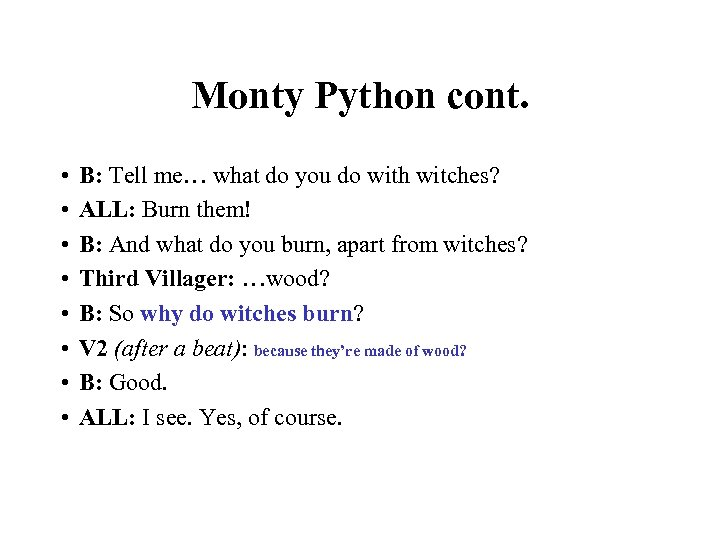Monty Python cont. • • B: Tell me… what do you do with witches? ALL: Burn them! B: And what do you burn, apart from witches? Third Villager: …wood? B: So why do witches burn? V 2 (after a beat): because they’re made of wood? B: Good. ALL: I see. Yes, of course.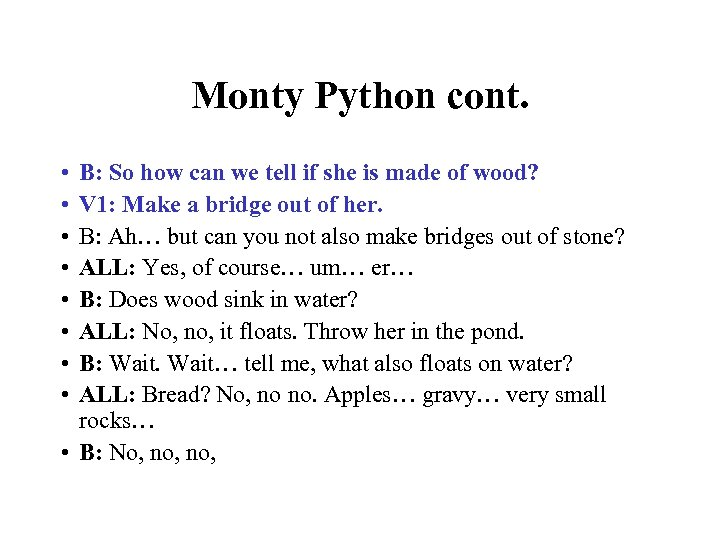Monty Python cont. • • B: So how can we tell if she is made of wood? V 1: Make a bridge out of her. B: Ah… but can you not also make bridges out of stone? ALL: Yes, of course… um… er… B: Does wood sink in water? ALL: No, no, it floats. Throw her in the pond. B: Wait… tell me, what also floats on water? ALL: Bread? No, no no. Apples… gravy… very small rocks… • B: No, no,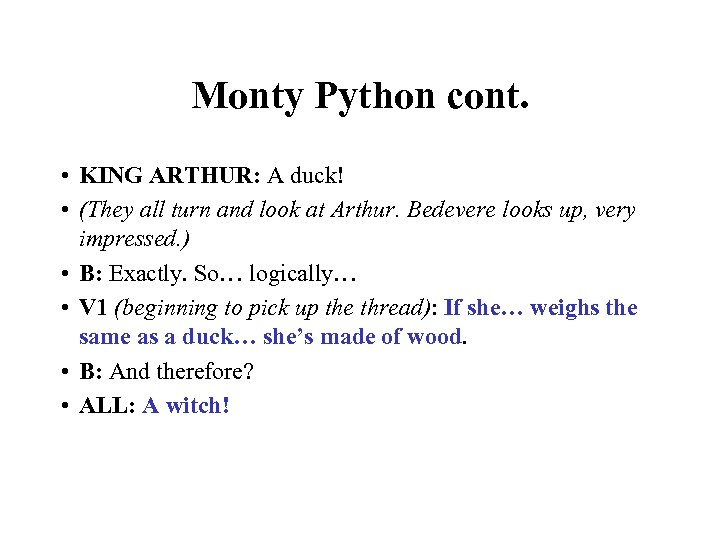Monty Python cont. • KING ARTHUR: A duck! • (They all turn and look at Arthur. Bedevere looks up, very impressed. ) • B: Exactly. So… logically… • V 1 (beginning to pick up the thread): If she… weighs the same as a duck… she’s made of wood. • B: And therefore? • ALL: A witch!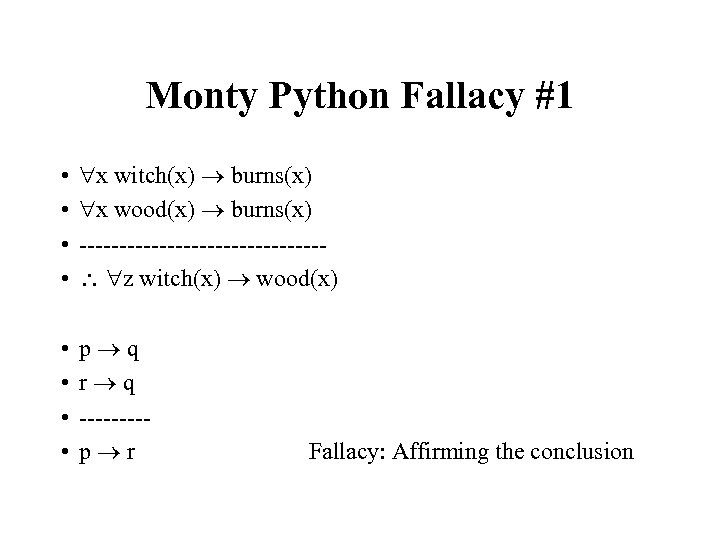Monty Python Fallacy #1 • • x witch(x) burns(x) x wood(x) burns(x) --------------- z witch(x) wood(x) • • p q r q ----p r Fallacy: Affirming the conclusion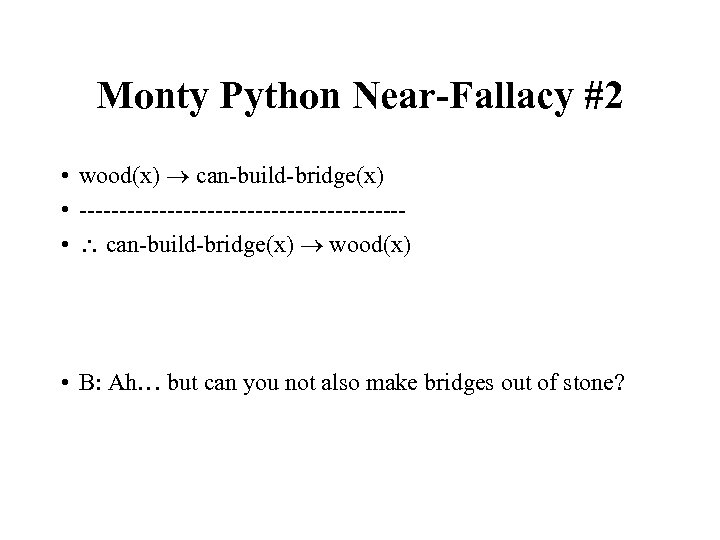Monty Python Near-Fallacy #2 • wood(x) can-build-bridge(x) • -------------------- • can-build-bridge(x) wood(x) • B: Ah… but can you not also make bridges out of stone?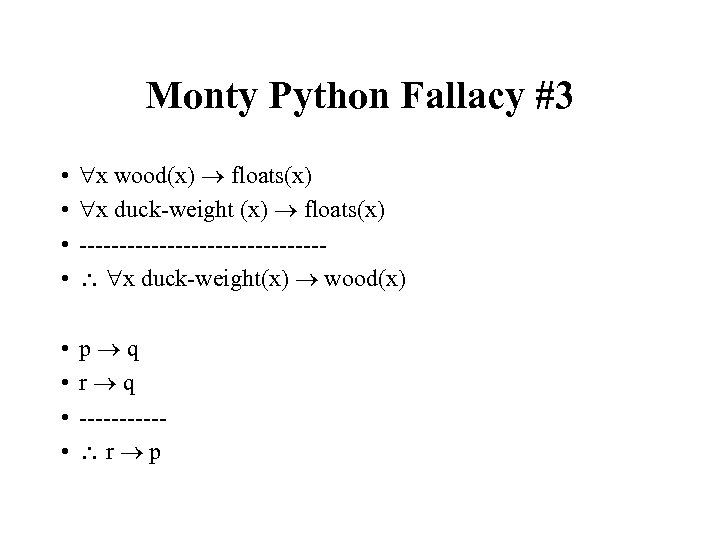Monty Python Fallacy #3 • • x wood(x) floats(x) x duck-weight (x) floats(x) --------------- x duck-weight(x) wood(x) • • p q r q ----- r p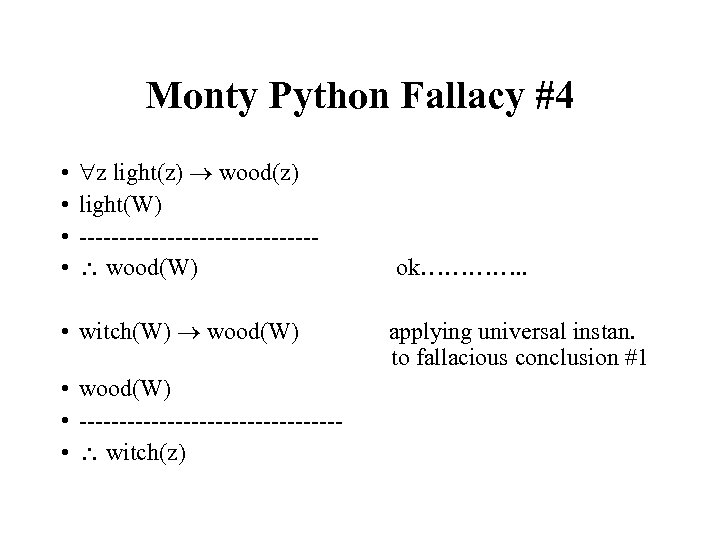Monty Python Fallacy #4 • • z light(z) wood(z) light(W) --------------- wood(W) • witch(W) wood(W) • ---------------- • witch(z) ok…………. . applying universal instan. to fallacious conclusion #1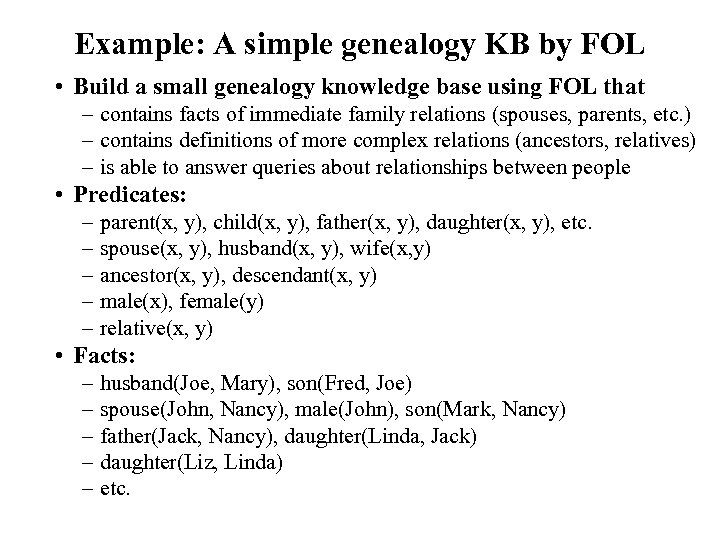Example: A simple genealogy KB by FOL • Build a small genealogy knowledge base using FOL that – contains facts of immediate family relations (spouses, parents, etc. ) – contains definitions of more complex relations (ancestors, relatives) – is able to answer queries about relationships between people • Predicates: – parent(x, y), child(x, y), father(x, y), daughter(x, y), etc. – spouse(x, y), husband(x, y), wife(x, y) – ancestor(x, y), descendant(x, y) – male(x), female(y) – relative(x, y) • Facts: – husband(Joe, Mary), son(Fred, Joe) – spouse(John, Nancy), male(John), son(Mark, Nancy) – father(Jack, Nancy), daughter(Linda, Jack) – daughter(Liz, Linda) – etc.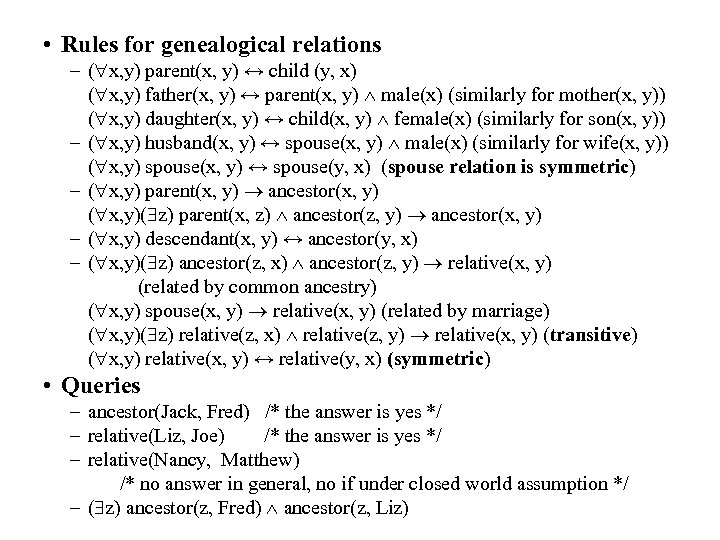• Rules for genealogical relations – ( x, y) parent(x, y) ↔ child (y, x) ( x, y) father(x, y) ↔ parent(x, y) male(x) (similarly for mother(x, y)) ( x, y) daughter(x, y) ↔ child(x, y) female(x) (similarly for son(x, y)) – ( x, y) husband(x, y) ↔ spouse(x, y) male(x) (similarly for wife(x, y)) ( x, y) spouse(x, y) ↔ spouse(y, x) (spouse relation is symmetric) – ( x, y) parent(x, y) ancestor(x, y) ( x, y)( z) parent(x, z) ancestor(z, y) ancestor(x, y) – ( x, y) descendant(x, y) ↔ ancestor(y, x) – ( x, y)( z) ancestor(z, x) ancestor(z, y) relative(x, y) (related by common ancestry) ( x, y) spouse(x, y) relative(x, y) (related by marriage) ( x, y)( z) relative(z, x) relative(z, y) relative(x, y) (transitive) ( x, y) relative(x, y) ↔ relative(y, x) (symmetric) • Queries – ancestor(Jack, Fred) /* the answer is yes */ – relative(Liz, Joe) /* the answer is yes */ – relative(Nancy, Matthew) /* no answer in general, no if under closed world assumption */ – ( z) ancestor(z, Fred) ancestor(z, Liz)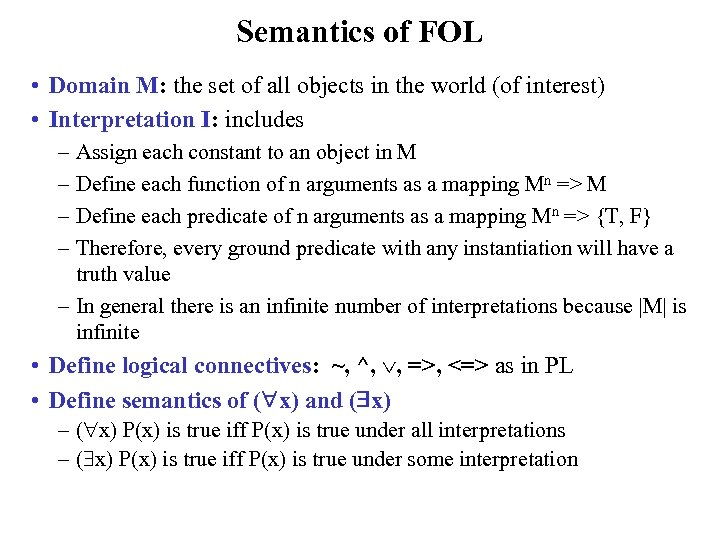Semantics of FOL • Domain M: the set of all objects in the world (of interest) • Interpretation I: includes – Assign each constant to an object in M – Define each function of n arguments as a mapping Mn => M – Define each predicate of n arguments as a mapping Mn => {T, F} – Therefore, every ground predicate with any instantiation will have a truth value – In general there is an infinite number of interpretations because |M| is infinite • Define logical connectives: ~, ^, , =>, <=> as in PL • Define semantics of ( x) and ( x) – ( x) P(x) is true iff P(x) is true under all interpretations – ( x) P(x) is true iff P(x) is true under some interpretation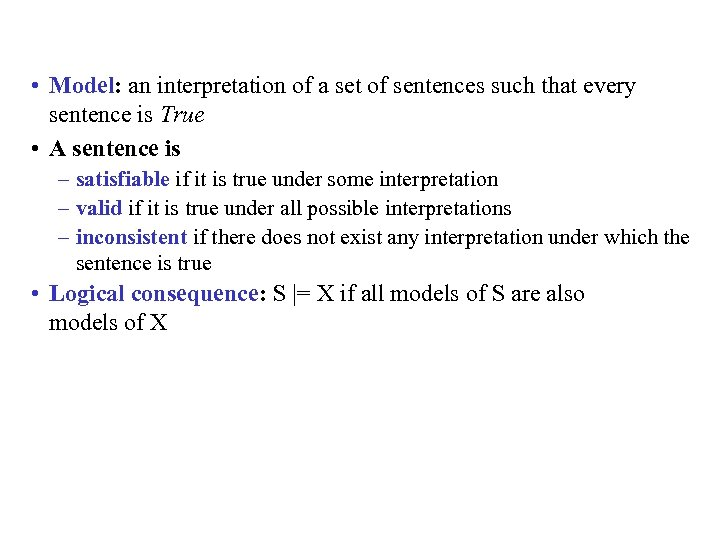• Model: an interpretation of a set of sentences such that every sentence is True • A sentence is – satisfiable if it is true under some interpretation – valid if it is true under all possible interpretations – inconsistent if there does not exist any interpretation under which the sentence is true • Logical consequence: S |= X if all models of S are also models of X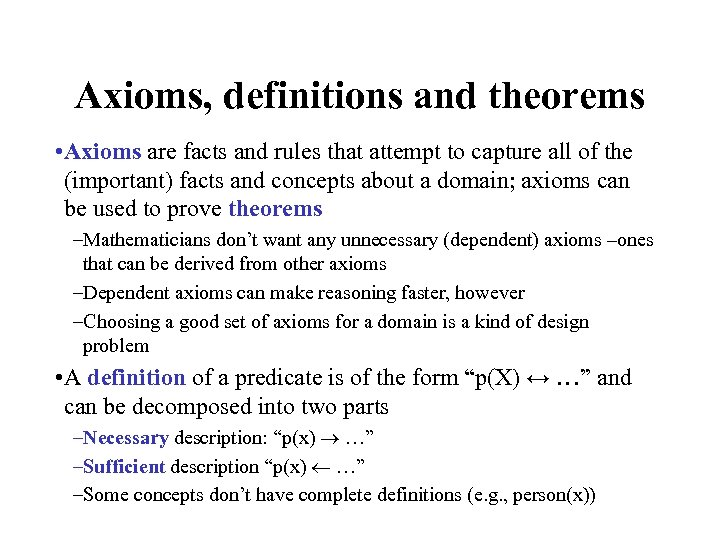Axioms, definitions and theorems • Axioms are facts and rules that attempt to capture all of the (important) facts and concepts about a domain; axioms can be used to prove theorems –Mathematicians don’t want any unnecessary (dependent) axioms –ones that can be derived from other axioms –Dependent axioms can make reasoning faster, however –Choosing a good set of axioms for a domain is a kind of design problem • A definition of a predicate is of the form “p(X) ↔ …” and can be decomposed into two parts –Necessary description: “p(x) …” –Sufficient description “p(x) …” –Some concepts don’t have complete definitions (e. g. , person(x))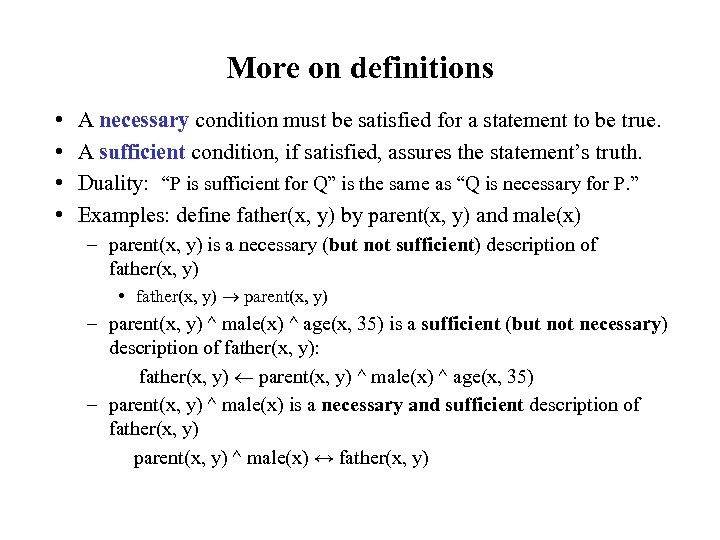More on definitions • • A necessary condition must be satisfied for a statement to be true. A sufficient condition, if satisfied, assures the statement’s truth. Duality: “P is sufficient for Q” is the same as “Q is necessary for P. ” Examples: define father(x, y) by parent(x, y) and male(x) – parent(x, y) is a necessary (but not sufficient) description of father(x, y) • father(x, y) parent(x, y) – parent(x, y) ^ male(x) ^ age(x, 35) is a sufficient (but not necessary) description of father(x, y): father(x, y) parent(x, y) ^ male(x) ^ age(x, 35) – parent(x, y) ^ male(x) is a necessary and sufficient description of father(x, y) parent(x, y) ^ male(x) ↔ father(x, y)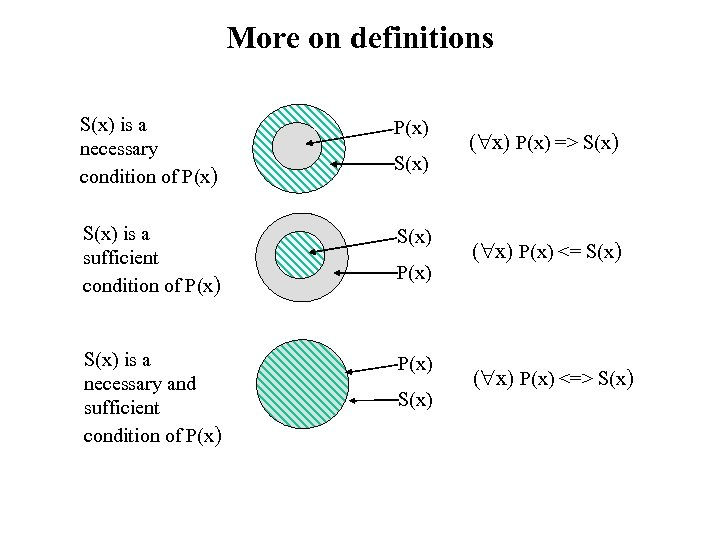More on definitions S(x) is a necessary condition of P(x) S(x) is a sufficient condition of P(x) S(x) is a necessary and sufficient condition of P(x) S(x) ( x) P(x) => S(x) ( x) P(x) <=> S(x)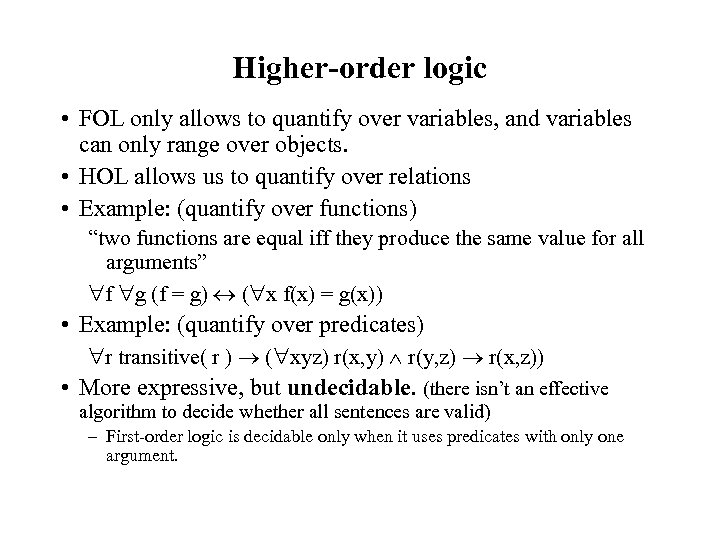Higher-order logic • FOL only allows to quantify over variables, and variables can only range over objects. • HOL allows us to quantify over relations • Example: (quantify over functions) “two functions are equal iff they produce the same value for all arguments” f g (f = g) ( x f(x) = g(x)) • Example: (quantify over predicates) r transitive( r ) ( xyz) r(x, y) r(y, z) r(x, z)) • More expressive, but undecidable. (there isn’t an effective algorithm to decide whether all sentences are valid) – First-order logic is decidable only when it uses predicates with only one argument.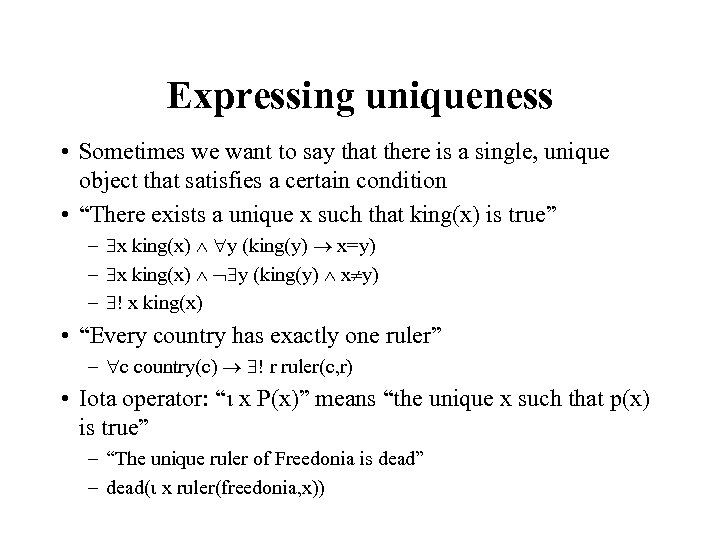Expressing uniqueness • Sometimes we want to say that there is a single, unique object that satisfies a certain condition • “There exists a unique x such that king(x) is true” – x king(x) y (king(y) x=y) – x king(x) y (king(y) x y) – ! x king(x) • “Every country has exactly one ruler” – c country(c) ! r ruler(c, r) • Iota operator: “ x P(x)” means “the unique x such that p(x) is true” – “The unique ruler of Freedonia is dead” – dead( x ruler(freedonia, x))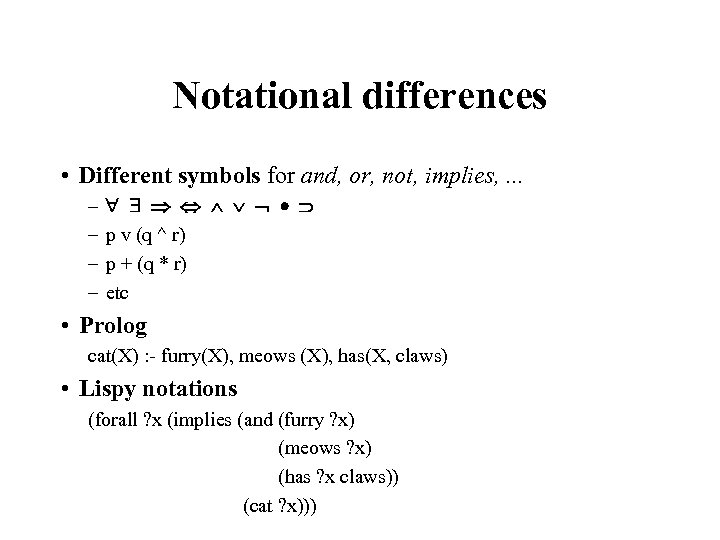Notational differences • Different symbols for and, or, not, implies, . . . – – p v (q ^ r) p + (q * r) etc • Prolog cat(X) : - furry(X), meows (X), has(X, claws) • Lispy notations (forall ? x (implies (and (furry ? x) (meows ? x) (has ? x claws)) (cat ? x)))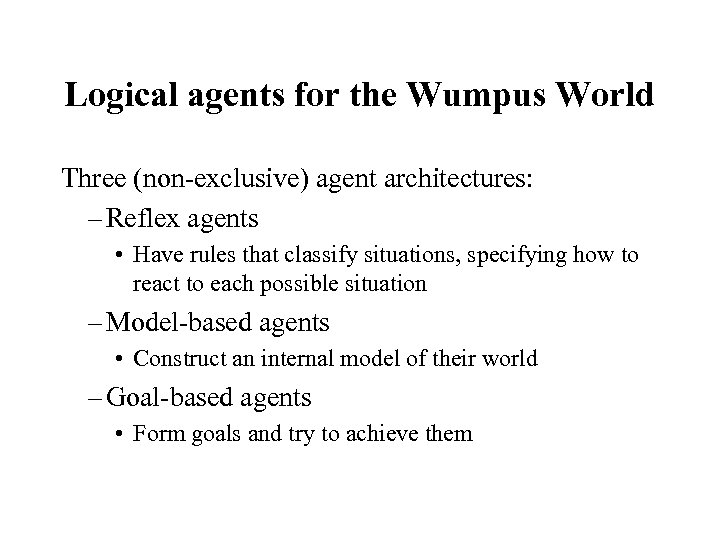Logical agents for the Wumpus World Three (non-exclusive) agent architectures: – Reflex agents • Have rules that classify situations, specifying how to react to each possible situation – Model-based agents • Construct an internal model of their world – Goal-based agents • Form goals and try to achieve them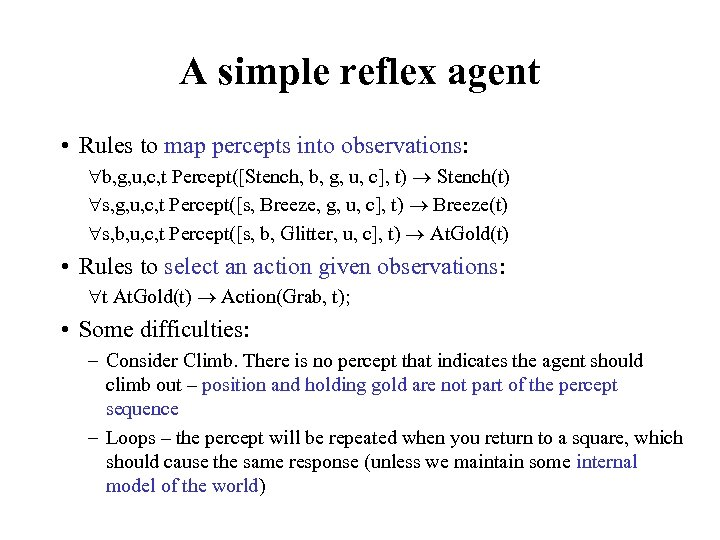A simple reflex agent • Rules to map percepts into observations: b, g, u, c, t Percept([Stench, b, g, u, c], t) Stench(t) s, g, u, c, t Percept([s, Breeze, g, u, c], t) Breeze(t) s, b, u, c, t Percept([s, b, Glitter, u, c], t) At. Gold(t) • Rules to select an action given observations: t At. Gold(t) Action(Grab, t); • Some difficulties: – Consider Climb. There is no percept that indicates the agent should climb out – position and holding gold are not part of the percept sequence – Loops – the percept will be repeated when you return to a square, which should cause the same response (unless we maintain some internal model of the world)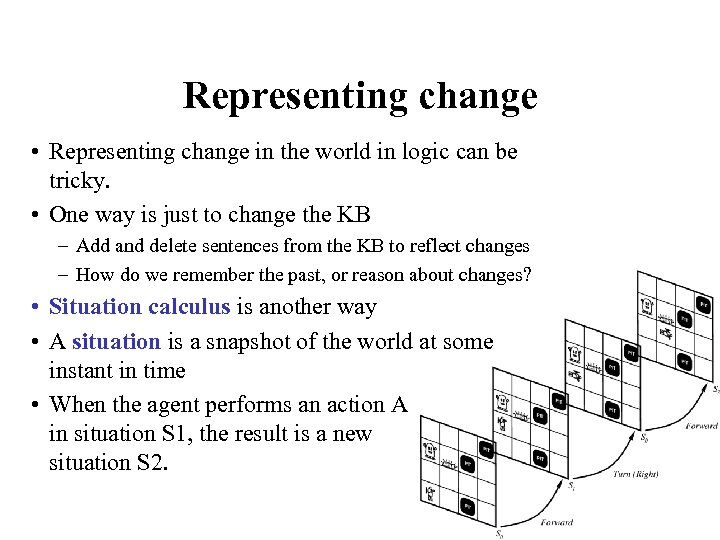Representing change • Representing change in the world in logic can be tricky. • One way is just to change the KB – Add and delete sentences from the KB to reflect changes – How do we remember the past, or reason about changes? • Situation calculus is another way • A situation is a snapshot of the world at some instant in time • When the agent performs an action A in situation S 1, the result is a new situation S 2.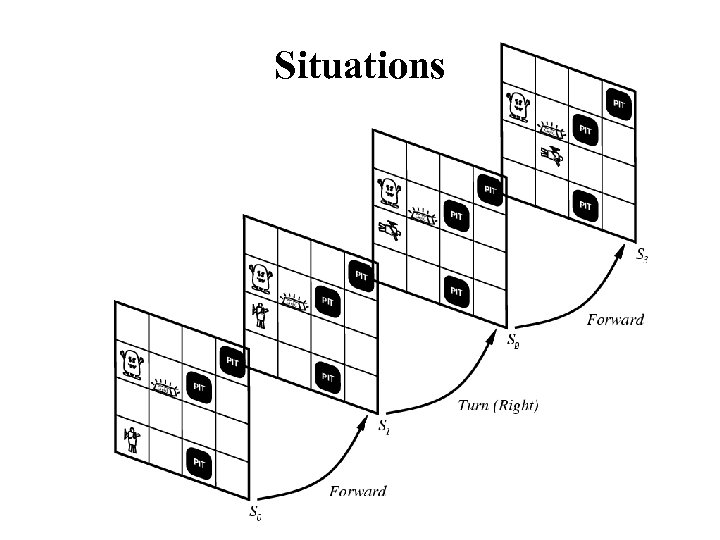Situations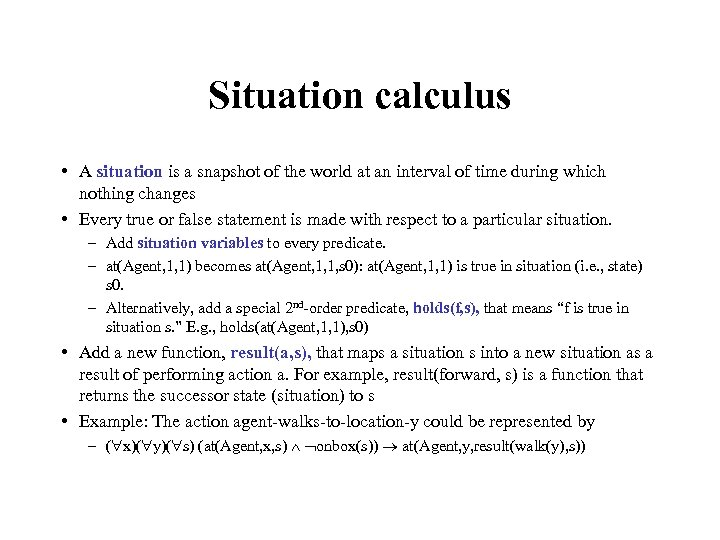Situation calculus • A situation is a snapshot of the world at an interval of time during which nothing changes • Every true or false statement is made with respect to a particular situation. – Add situation variables to every predicate. – at(Agent, 1, 1) becomes at(Agent, 1, 1, s 0): at(Agent, 1, 1) is true in situation (i. e. , state) s 0. – Alternatively, add a special 2 nd-order predicate, holds(f, s), that means “f is true in situation s. ” E. g. , holds(at(Agent, 1, 1), s 0) • Add a new function, result(a, s), that maps a situation s into a new situation as a result of performing action a. For example, result(forward, s) is a function that returns the successor state (situation) to s • Example: The action agent-walks-to-location-y could be represented by – ( x)( y)( s) (at(Agent, x, s) onbox(s)) at(Agent, y, result(walk(y), s))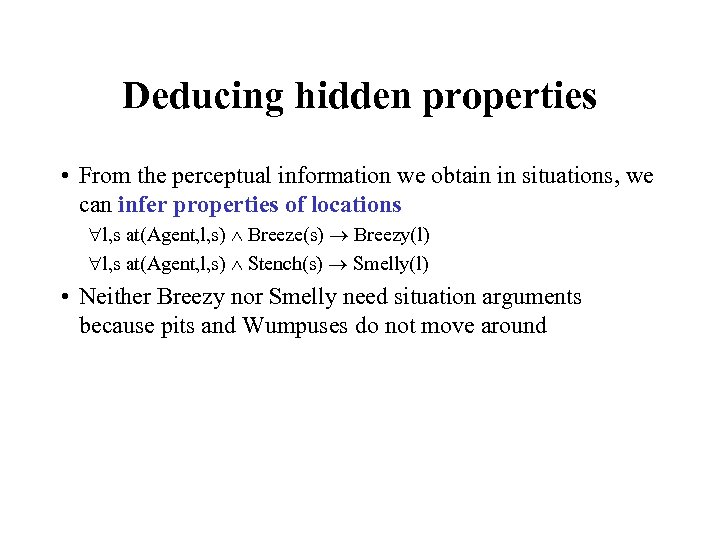Deducing hidden properties • From the perceptual information we obtain in situations, we can infer properties of locations l, s at(Agent, l, s) Breeze(s) Breezy(l) l, s at(Agent, l, s) Stench(s) Smelly(l) • Neither Breezy nor Smelly need situation arguments because pits and Wumpuses do not move around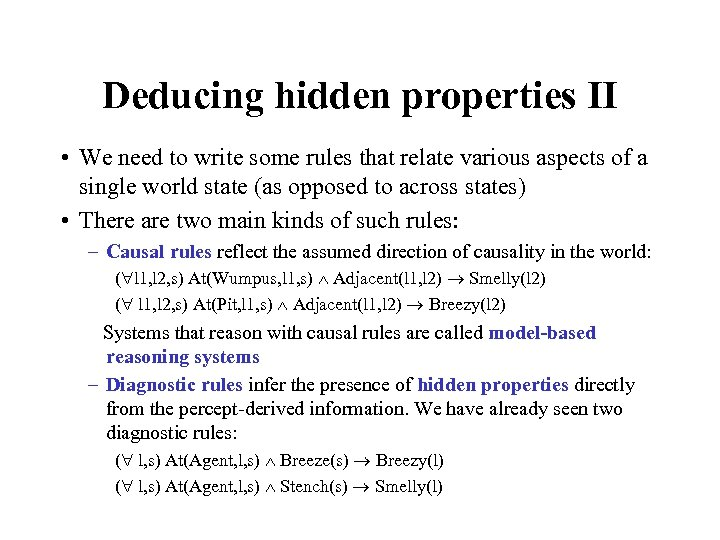Deducing hidden properties II • We need to write some rules that relate various aspects of a single world state (as opposed to across states) • There are two main kinds of such rules: – Causal rules reflect the assumed direction of causality in the world: ( l 1, l 2, s) At(Wumpus, l 1, s) Adjacent(l 1, l 2) Smelly(l 2) ( l 1, l 2, s) At(Pit, l 1, s) Adjacent(l 1, l 2) Breezy(l 2) Systems that reason with causal rules are called model-based reasoning systems – Diagnostic rules infer the presence of hidden properties directly from the percept-derived information. We have already seen two diagnostic rules: ( l, s) At(Agent, l, s) Breeze(s) Breezy(l) ( l, s) At(Agent, l, s) Stench(s) Smelly(l)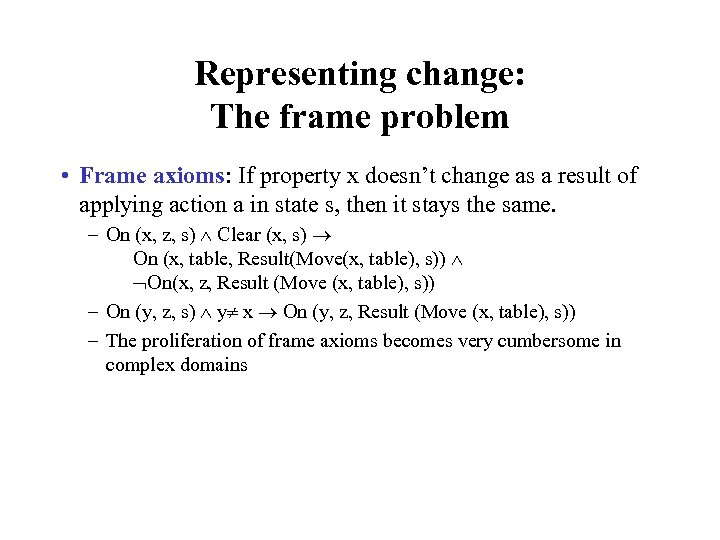Representing change: The frame problem • Frame axioms: If property x doesn’t change as a result of applying action a in state s, then it stays the same. – On (x, z, s) Clear (x, s) On (x, table, Result(Move(x, table), s)) On(x, z, Result (Move (x, table), s)) – On (y, z, s) y x On (y, z, Result (Move (x, table), s)) – The proliferation of frame axioms becomes very cumbersome in complex domains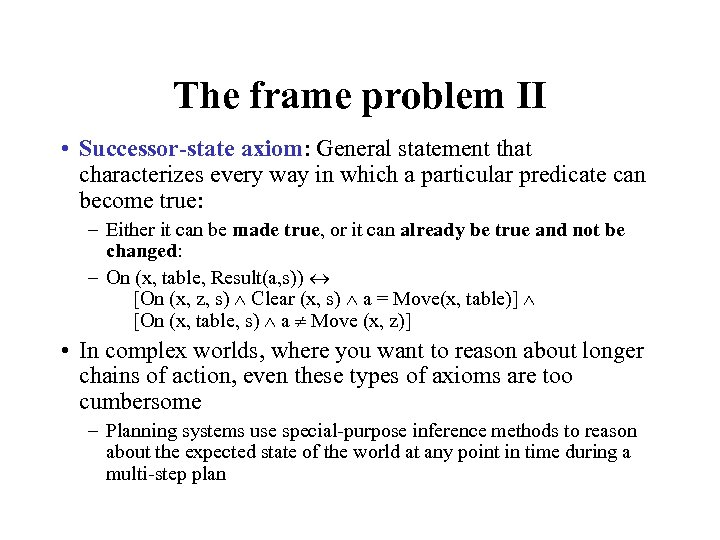The frame problem II • Successor-state axiom: General statement that characterizes every way in which a particular predicate can become true: – Either it can be made true, or it can already be true and not be changed: – On (x, table, Result(a, s)) [On (x, z, s) Clear (x, s) a = Move(x, table)] [On (x, table, s) a Move (x, z)] • In complex worlds, where you want to reason about longer chains of action, even these types of axioms are too cumbersome – Planning systems use special-purpose inference methods to reason about the expected state of the world at any point in time during a multi-step plan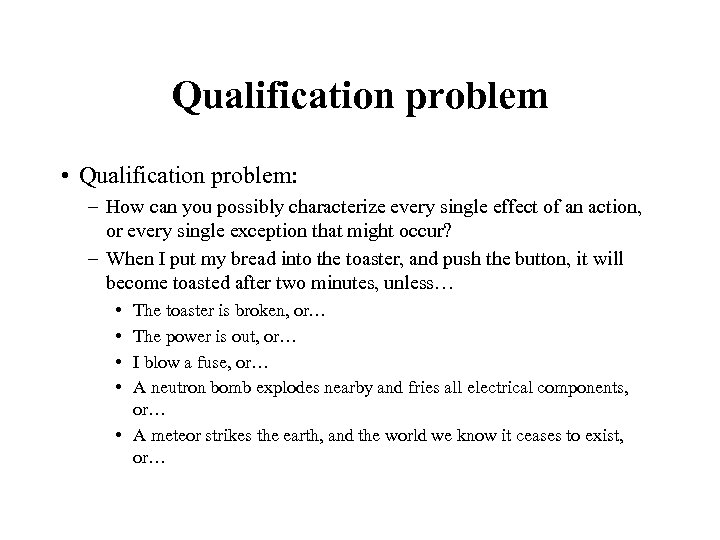Qualification problem • Qualification problem: – How can you possibly characterize every single effect of an action, or every single exception that might occur? – When I put my bread into the toaster, and push the button, it will become toasted after two minutes, unless… • • The toaster is broken, or… The power is out, or… I blow a fuse, or… A neutron bomb explodes nearby and fries all electrical components, or… • A meteor strikes the earth, and the world we know it ceases to exist, or…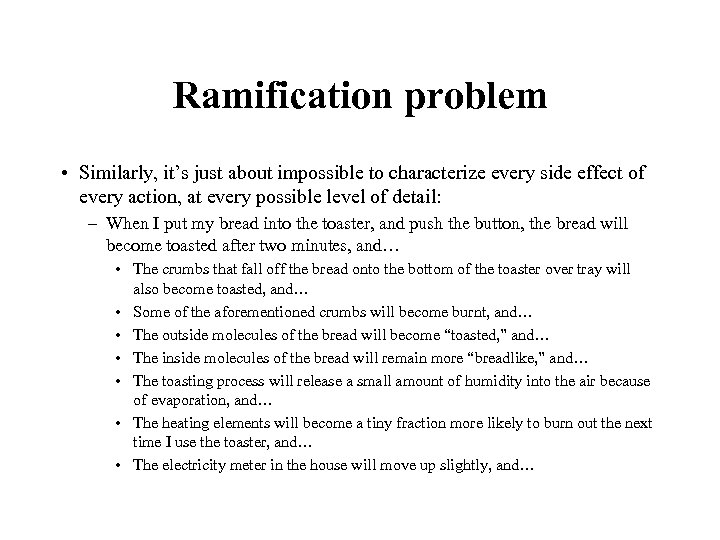Ramification problem • Similarly, it’s just about impossible to characterize every side effect of every action, at every possible level of detail: – When I put my bread into the toaster, and push the button, the bread will become toasted after two minutes, and… • The crumbs that fall off the bread onto the bottom of the toaster over tray will also become toasted, and… • Some of the aforementioned crumbs will become burnt, and… • The outside molecules of the bread will become “toasted, ” and… • The inside molecules of the bread will remain more “breadlike, ” and… • The toasting process will release a small amount of humidity into the air because of evaporation, and… • The heating elements will become a tiny fraction more likely to burn out the next time I use the toaster, and… • The electricity meter in the house will move up slightly, and…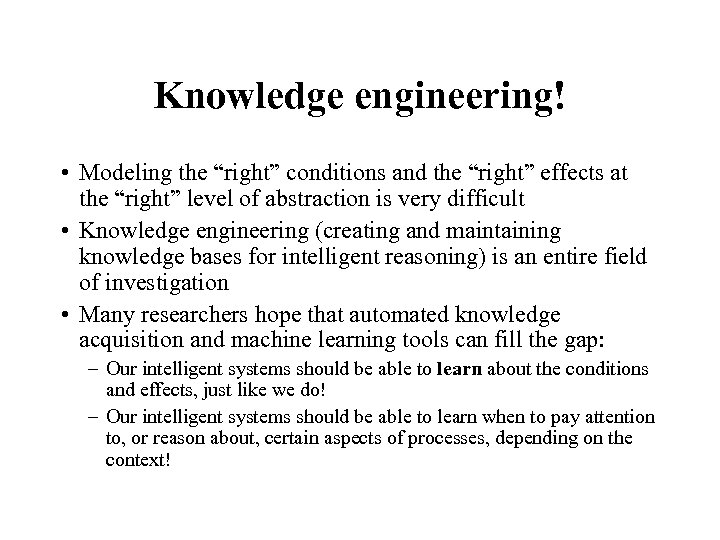Knowledge engineering! • Modeling the “right” conditions and the “right” effects at the “right” level of abstraction is very difficult • Knowledge engineering (creating and maintaining knowledge bases for intelligent reasoning) is an entire field of investigation • Many researchers hope that automated knowledge acquisition and machine learning tools can fill the gap: – Our intelligent systems should be able to learn about the conditions and effects, just like we do! – Our intelligent systems should be able to learn when to pay attention to, or reason about, certain aspects of processes, depending on the context!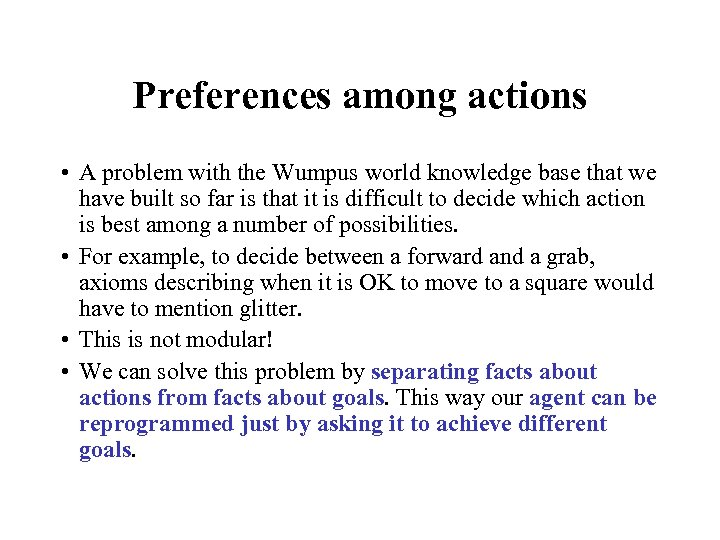Preferences among actions • A problem with the Wumpus world knowledge base that we have built so far is that it is difficult to decide which action is best among a number of possibilities. • For example, to decide between a forward and a grab, axioms describing when it is OK to move to a square would have to mention glitter. • This is not modular! • We can solve this problem by separating facts about actions from facts about goals. This way our agent can be reprogrammed just by asking it to achieve different goals.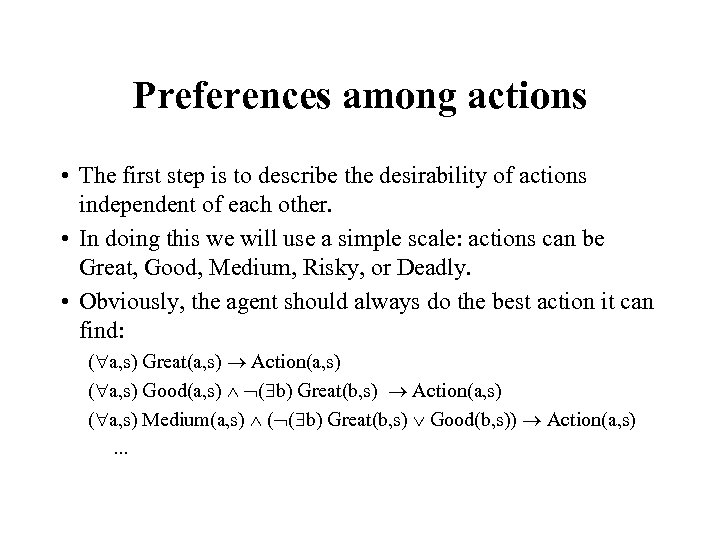Preferences among actions • The first step is to describe the desirability of actions independent of each other. • In doing this we will use a simple scale: actions can be Great, Good, Medium, Risky, or Deadly. • Obviously, the agent should always do the best action it can find: ( a, s) Great(a, s) Action(a, s) ( a, s) Good(a, s) ( b) Great(b, s) Action(a, s) ( a, s) Medium(a, s) ( ( b) Great(b, s) Good(b, s)) Action(a, s). . .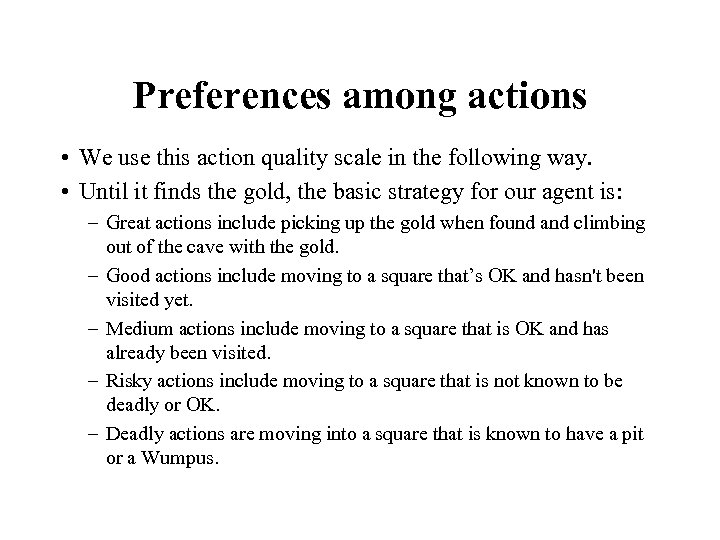Preferences among actions • We use this action quality scale in the following way. • Until it finds the gold, the basic strategy for our agent is: – Great actions include picking up the gold when found and climbing out of the cave with the gold. – Good actions include moving to a square that’s OK and hasn't been visited yet. – Medium actions include moving to a square that is OK and has already been visited. – Risky actions include moving to a square that is not known to be deadly or OK. – Deadly actions are moving into a square that is known to have a pit or a Wumpus.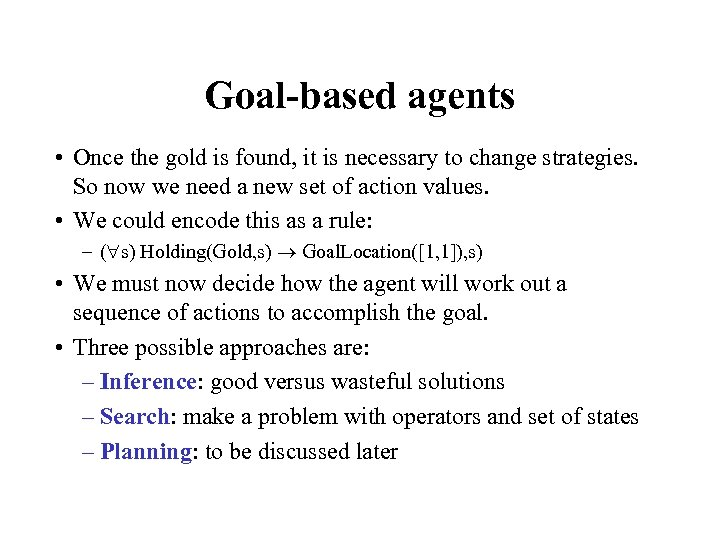Goal-based agents • Once the gold is found, it is necessary to change strategies. So now we need a new set of action values. • We could encode this as a rule: – ( s) Holding(Gold, s) Goal. Location([1, 1]), s) • We must now decide how the agent will work out a sequence of actions to accomplish the goal. • Three possible approaches are: – Inference: good versus wasteful solutions – Search: make a problem with operators and set of states – Planning: to be discussed later